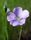## How to trace source datasets in the proc sql output?

How to trace source datasets in the proc sql output?

My goal is to figure out the contribution of missings, say, for variable "latitude". I'm a novice to CASE WHEN and COALESCE functions in proc sql. Therefore, proc sql code below is to rather explain how I envisioned what might have worked out. There are nice SUGI papers but I didn't find one that discussed about indicator variable in "both_missing" scenario.

``````data data1;
input id latitude;
cards;
1001 43.2
1003 43.6
1004 .
;

DATA data2;
INPUT id latitude;
CARDS;
1001 43.2
1002 48.3
1004 .
;

data want;
input id_a id_b lat_a lat_b indicator \$;
cards;
1001 1001 43.2 43.2	match
.	 1002 .    48.3	a_miss
1003 .	  43.6 .	b_miss
1004 1004 .    .	both_miss
;

PROC SQL;
CREATE TABLE want AS
SELECT a.id as id_a,
a.latitude as lat_a,
b.id as id_b,
b.latitude as lat_b,
CASE (a.latitude = b.latitude)
WHEN lat_a=lat_b THEN 'Match'
WHEN lat_a=. THEN 'a_miss'
WHEN lat_b=. THEN 'b_miss'
WHEN lat_a=. and lat_b=. THEN 'both_miss'
ELSE 'else'
END
AS indvar LENGTH=5
FROM data1 a join data2 b
ON a.id = b.id;
QUIT;
``````

1 ACCEPTED SOLUTION

Accepted SolutionsKsharp
Super User

## Re: How to trace source datasets in the proc sql output?

``````data data1;
input id latitude;
cards;
1001 43.2
1003 43.6
1004 .
;

DATA data2;
INPUT id latitude;
CARDS;
1001 43.2
1002 48.3
1004 .
;

proc sql;
create table want as
select a.id as id_a,b.id as id_b,
a.latitude as lat_a,b.latitude as lat_b
from data1 as a full join data2 as b
on a.id=b.id;
quit;``````
6 REPLIES 6

## Re: How to trace source datasets in the proc sql output?

Are you trying to replicate the IN functionality of a data step merge?LinusH
Tourmaline | Level 20

## Re: How to trace source datasets in the proc sql output?

The current syntax is incorrect, the condition immediately after CASE needs to be removed.

Also, SQL evaluates WHEN in sequence, therefore you need to move the "both" upwards in the code.

Also, I think you need to define lat_a given your input program.

But haven't you test your code? Do that and return with a more specific question...

Data never sleepsKsharp
Super User

## Re: How to trace source datasets in the proc sql output?

It is very easy for Data Step.

``````data data1;
input id latitude;
cards;
1001 43.2
1003 43.6
1004 .
;

DATA data2;
INPUT id latitude;
CARDS;
1001 43.2
1002 48.3
1004 .
;

data want;
merge data1(in=ina rename=(latitude=latitude_a))
data2(in=inb rename=(latitude=latitude_b));
by id;
if ina then id_a=id;
if inb then id_b=id;
drop id;
run;``````

## Re: How to trace source datasets in the proc sql output?

I have achieved it in data step. I was wondering whether proc sql has a way to achieve the result similarly?Ksharp
Super User

## Re: How to trace source datasets in the proc sql output?

``````data data1;
input id latitude;
cards;
1001 43.2
1003 43.6
1004 .
;

DATA data2;
INPUT id latitude;
CARDS;
1001 43.2
1002 48.3
1004 .
;

proc sql;
create table want as
select a.id as id_a,b.id as id_b,
a.latitude as lat_a,b.latitude as lat_b
from data1 as a full join data2 as b
on a.id=b.id;
quit;``````

## Re: How to trace source datasets in the proc sql output?

Summarizing from responses:

proc sql:

``````proc sql;
create table sharp as
select a.id as id_a,b.id as id_b,
a.latitude as lat_a,b.latitude as lat_b
from data1 as a full join data2 as b
on a.id=b.id;
quit;

data sharp1; set sharp;
length source \$ 9;
if lat_a ^=. then source='a';
if lat_b ^=. then source='b';
if lat_a =. and lat_b=. then source='both_miss';
if lat_a ^=. and lat_b^=. then source='match';
run;``````
``proc sort data=first;by uid;proc sort data=second;by uid;data check; merge first (in=ina rename=(latitude=lat_a)) second(in=inb rename=(latitude=lat_b)); by uid; if ina then id_a=uid; if inb then id_b=uid; drop uid; if lat_a=. then first=.; if lat_b=. then second=.; if lat_a^=. then first=1; if lat_b^=. then second=1;run;proc freq data=m.check;tables first*second;run;``

Discussion stats
• 6 replies
• 918 views
• 1 like
• 4 in conversation